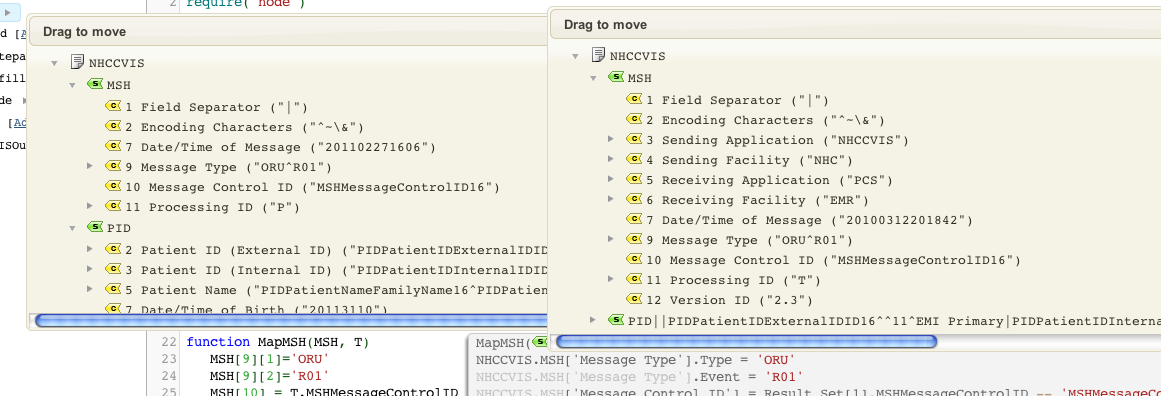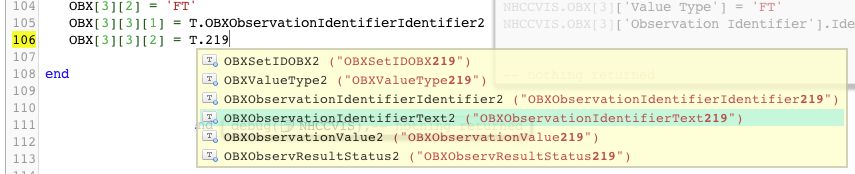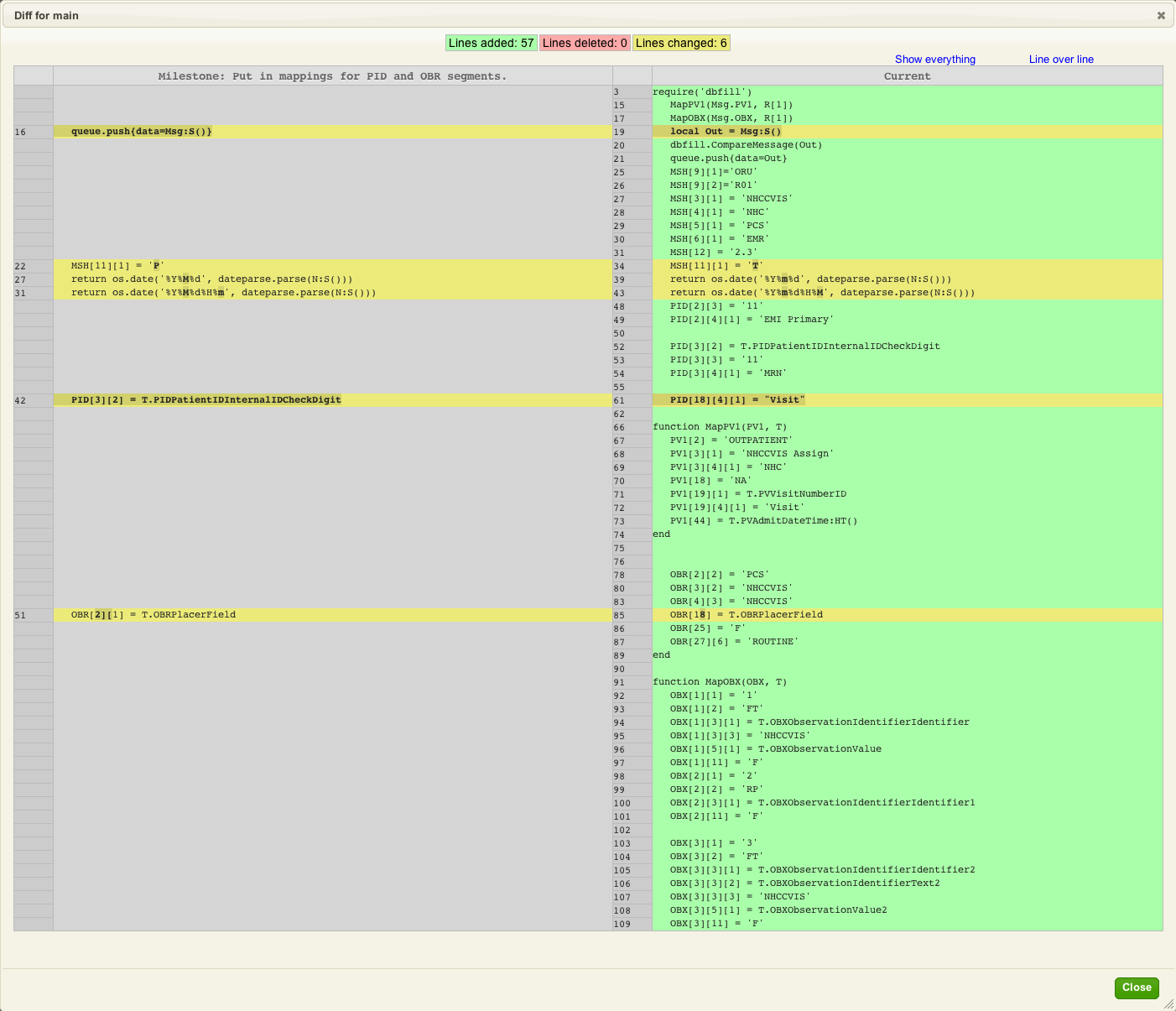# Database to HL7

## Contents

So I added ‘dbfill’ to the filter routine and added a new routine called dbfill with this code:

```function dbfill.CompareMessage(Data)
local Out = hl7.parse{vmd='CVISOutbound.vmd', data=Data}
end```

And then tweaked the main routine to call this code:

```local Out = Msg:S()
dbfill.CompareMessage(Out)
queue.push{data=Out}```

That told me my first problem with the new mapping code. It was missing the message type! Oops.

Easy enough to solve with two additional lines of script (on lines 23 and 24) in the MapMSH function:Then I altered the dbfill routine to:

```function dbfill.LoadMessage(Base, Msg)
local FileName = 'D:temp'..Base
..Msg.MSH..'.txt'
trace(FileName)
local F = io.open(FileName, "r")
end

function dbfill.CompareMessage(Data)
local Out = hl7.parse{vmd='CVISOutbound.vmd', data=Data}
return hl7.parse{vmd='CVISOutbound.vmd', data=Orig}
end```

This is where the real power of the Translator shows through because we are returning a parsed node tree from the loading routine we can open up parallel dialogs for the original message and the new output message and compare them along side each other. This screen shot shows exactly that with the left hand side showing the new tree and the right hand side the original output:It’s easy to see the gaps that we have to fill in the new interface. I went through and added in the missing MSH fields:

```function MapMSH(MSH, T)
MSH='ORU'
MSH='R01'
MSH = 'NHCCVIS'
MSH = 'NHC'
MSH = 'PCS'
MSH = 'EMR'
MSH = '2.3'
MSH = T.MSHMessageControlID
MSH = os.date('%Y%M%d%H%m')
MSH = 'T'
return MSH
end```

I went through the script putting in the mappings. Some observations:

1. Having very regularly named source data made it quite easy see if data was set as a constant or came from the database.
2. Deep auto-completion was very helpful in locating source data in the database table to map it.

To give an example see:You can see in the above I was searching for the key phrase “219” in order to find the OBXObservationIdentifierText219 value.

Doing things this way makes for a very efficient work flow. It took me about 1/2 hour to flesh out the rest of the mappings. My script looked like this now:

```require 'dateparse'
require 'node'
require 'dbfill'

local conn = db.connect{
api=db.SQLITE,
name='test',
live=true
}

local function trace(a,b,c,d,) return end

function main(Data)
local R = conn:query{
live=true,
sql="SELECT * FROM NHCCVISREPORT WHERE MESSAGE_ID = "..Data
}

local Msg = hl7.message{vmd='CVISOutbound.vmd', name='NHCCVIS'}

MapMSH(Msg.MSH, R)
MapPID(Msg.PID, R)
MapPV1(Msg.PV1, R)
MapOBR(Msg.OBR, R)
MapOBX(Msg.OBX, R)
trace(Msg)
local Out = Msg:S()
dbfill.CompareMessage(Out)
queue.push{data=Out}
end

function MapMSH(MSH, T)
MSH='ORU'
MSH='R01'
MSH = 'NHCCVIS'
MSH = 'NHC'
MSH = 'PCS'
MSH = 'EMR'
MSH = '2.3'
MSH = T.MSHMessageControlID
MSH = os.date('%Y%M%d%H%m')
MSH = 'T'
return MSH
end

function node.HD(N)
return os.date('%Y%m%d', dateparse.parse(N:S()))
end

function node.HT(N)
return os.date('%Y%m%d%H%M', dateparse.parse(N:S()))
end

function MapPID(PID, T)
PID = T.PIDPatientIDExternalIDID
PID = '11'
PID = 'EMI Primary'

PID = T.PIDPatientIDInternalIDID
PID = T.PIDPatientIDInternalIDCheckDigit
PID = '11'
PID = 'MRN'

PID = T.PIDPatientNameFamilyName
PID = T.PIDPatientNameGivenName
PID = T.PIDDateTimeofBirth:HD()
PID = T.PIDSex
PID = T.PIDPatientAccountNumberID
PID = "Visit"

return PID
end

function MapPV1(PV1, T)
PV1 = 'OUTPATIENT'
PV1 = 'NHCCVIS Assign'
PV1 = 'NHC'
PV1 = 'NA'
PV1 = T.PVVisitNumberID
PV1 = 'Visit'
end

function MapOBR(OBR, T)
OBR = 'PCS'
OBR = T.OBRFillerOrderNumberEntityIdentifier
OBR = 'NHCCVIS'
OBR = T.OBRUniversalServiceIDIdentifier
OBR = T.OBRUniversalServiceIDText
OBR = 'NHCCVIS'
OBR = T.OBRObservationDateTime:HT()
OBR = T.OBRPlacerField
OBR = 'F'
OBR = 'ROUTINE'
return OBR
end

function MapOBX(OBX, T)
OBX = '1'
OBX = 'FT'
OBX = T.OBXObservationIdentifierIdentifier
OBX = 'NHCCVIS'
OBX = T.OBXObservationValue
OBX = 'F'
OBX = '2'
OBX = 'RP'
OBX = T.OBXObservationIdentifierIdentifier1
OBX = 'F'

OBX = '3'
OBX = 'FT'
OBX = T.OBXObservationIdentifierIdentifier2
OBX = T.OBXObservationIdentifierText2
OBX = 'NHCCVIS'
OBX = T.OBXObservationValue2
OBX = 'F'
end```

This screen shot shows the changes as we can see inside the editor, you can really see the power of having integrated version control in the Translator:## Next Step?

At this point our equivalent mapping is fairly complete. What is required now is to use the power of the Translator to programmatically identify the last few remaining differences between the old implementation of the interface and the new one.